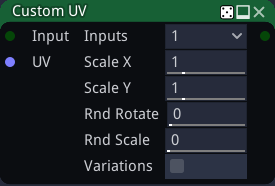# CustomUV node¶

The CustomUV node deforms an input image according to a custom UV map given as input.## Inputs¶

The CustomUV node accepts two inputs:

• The Source inputs is the image to be deformed.

• The UV input is a color image whose red and green channels are used as U and V (X and Y in texture space) coordinates, and the blue channel holds a value to be used for pseudo-random scale and rotate transforms.

## Outputs¶

The CustomUV node outputs the deformed image.

## Parameters¶

The CustomUV node accepts the following parameters:

• Inputs is the number of alternate shapes in the input (1, 4 or 16). Images containing several shapes can easily be created using the Tile2x2 node.

• Scale X and Scale Y are the scale along X and Y axes applied to each instance.

• Rnd Rotate is the maximum angle of the random rotation applied to each instance.

• Rnd Scale is the amount of random scaling applied to each instance.

• Variations: if checked, the node will deform different variations of its input (i.e. roll a different seed for each instance)